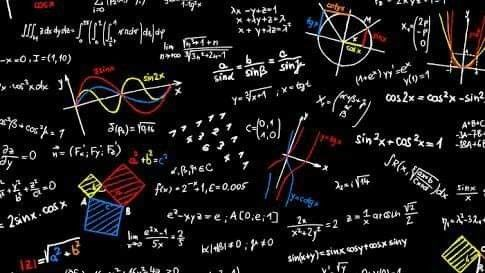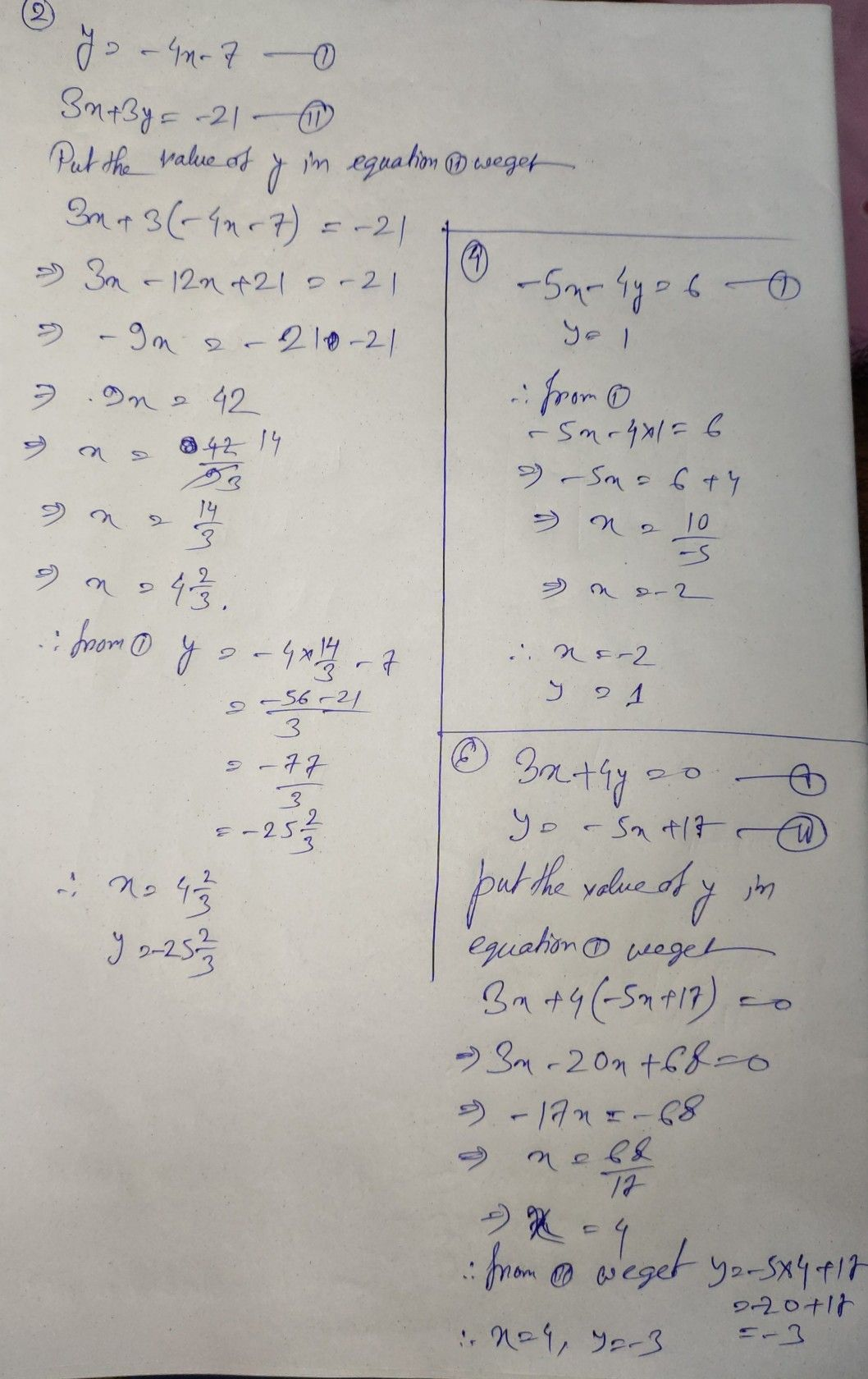Symbol
Problem$2\right)$ $y=-4x-7$ $3x+3y=-21$ $4\right)$ $-5x-4y=6$ $y=1$ $6\right)$ $3x+41=0$ $=-5x+17$